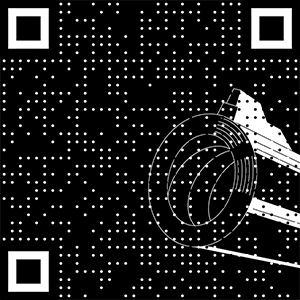# 面试高频考题——手撸一个 LRU 算法！

2021/05/12 08:46

LRU 就是 Least Recently Used 的缩写，翻译过来就是“最近最少使用”。 也就是说 LRU 算法会将最近最少用的缓存移除，让给最新使用的缓存。这是非常常见的一个缓存淘汰策略

class Node {    int key;    int value;    Node prev;    Node next;    Node() {    }    Node(int key, int value) {        this.key = key;        this.value = value;    }}

class LRUCache {    class Node {        int key;        int value;        Node prev;        Node next;        Node() {        }        Node(int key, int value) {            this.key = key;            this.value = value;        }    }    private int size;    private int capacity;    private Map<Integer, Node> cache;    /* 虚拟头节点 */    private Node head;    /* 虚拟尾节点 */    private Node tail;    public LRUCache(int capacity) {        this.size = 0;        this.capacity = capacity;        cache = new HashMap<>();        head = new Node();        tail = new Node();        head.next = tail;        tail.prev = head;    }    public int get(int key) {        Node node = cache.get(key);        if (node == null) {            return -1;        }        // 将最近这次访问的数据移到头部        moveToHead(node);        return node.value;    }    public void put(int key, int value) {        Node node = cache.get(key);        if (node == null) {            Node newNode = new Node(key, value);            cache.put(key, newNode);            // 将最近新增的数据放到头部            addToHead(newNode);            ++size;            // 若数据量超过设定的最大容量，移除尾部（最不常访问）的节点数据            if (size > capacity) {                Node tail = removeTail();                // 缓存淘汰，移除尾部节点数据                cache.remove(tail.key);                --size;            }        } else {            node.value = value;            moveToHead(node);        }    }    private void moveToHead(Node node) {        removeNode(node);        addToHead(node);    }    private void removeNode(Node node) {        node.prev.next = node.next;        node.next.prev = node.prev;    }    private void addToHead(Node node) {        node.next = head.next;        head.next = node;        node.next.prev = node;        node.prev = head;    }    private Node removeTail() {        Node node = tail.prev;        removeNode(node);        return node;    }}### 作者的其它热门文章

0
0 收藏

0 评论
0 收藏
0